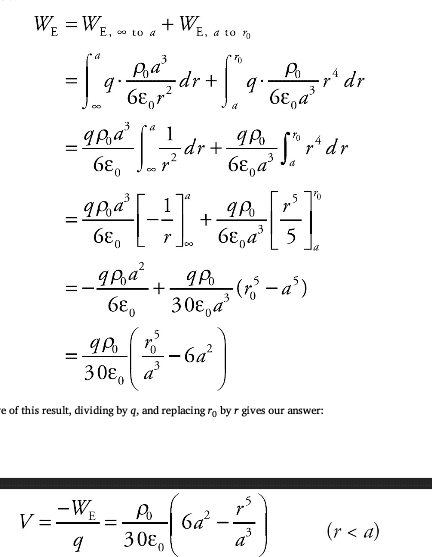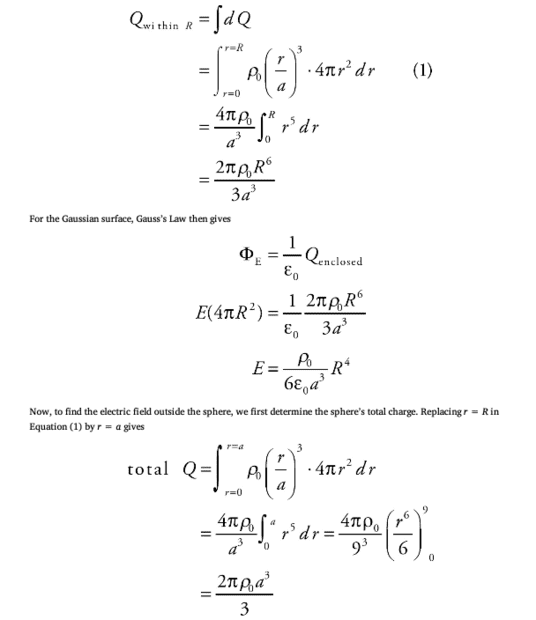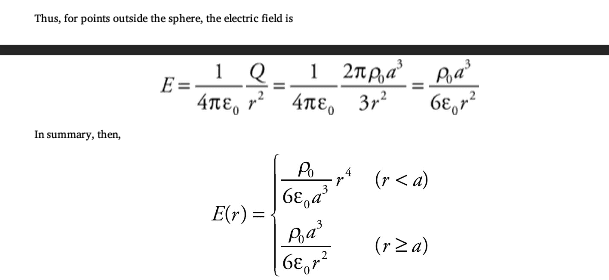# Find the E-field and Potential for a solid, nonconducting charged sphere

L_ucifer
Homework Statement:
A solid, nonconducting sphere of radius a has a volume charge density given by the equation ρ(r) = ρ0(r/a)^3, where r is the distance from the sphere’s centre.
(a) Determine the electric field magnitude, E(r), as a function of r.
(b) Determine the potential, V(r), as a function of r. Take the zero of potential at r = ∞.
Relevant Equations:
E = -dV/dr
V = -W/q
I understand part (a) of this question, and my answer for that part is:

*For r < a*
E = (ρ0 * r4) / (6 * ε0 * a3)
* For r ≥ a*
E = (ρ0 * a3) / (6 * ε0 * r2)

Now, for part (b), I understand one solution is, for r < a, find the work done to bring a point charge q from infinity to a and then from a to r and divide the work by q. This solution is attached below:Now, we also know that E = -dV/dr. This checks out because when we take the negative derivative of V found in the solution, we get the equation for E when r < a. This derivative can also be written as: V = -∫E*dr; however, when I integrate the equation of E for r < a, I get a different solution. Specifically, my solution is the same as the given solution except it doesn't have 6 as a coefficient for a2. Is there a gap in my understanding? What have I done wrong? I appreciate the support.

EDIT: Updated the variables and fixed the typesetting.

Last edited:

Staff Emeritus
Homework Helper
Gold Member
I understand part (a) of this question, and my answer for that part is:

*For r < a*
E = (ρ0 * r4) / (6 * ε0 * a3)
* For r ≥ a*
E = (ρ0 * R3) / (6 * ε0 * r2)

L_ucifer
I apologise, I meant E = (ρ0 * a3) / (6 * ε0 * r2) for r ≥ aStaff Emeritus
Homework Helper
Gold Member
Still incorrect with the problem as stated. You are not using the density quoted in the problem statement.

Homework Helper
Gold Member
Still incorrect with the problem as stated. You are not using the density quoted in the problem statement.
I got the same fields as OP with ##\rho(r)=\rho_0\left(\dfrac{r}{a}\right)^3##. Maybe the discrepancy is due to careless typesetting in the statement of the problem in which the radius of the distribution appears ##R## instead of ##a##. The ratio ##r/a## is cubed in the solution, not multiplied by 3.

L_ucifer
I got the same fields as OP with ##\rho(r)=\rho_0\left(\dfrac{r}{a}\right)^3##. Maybe the discrepancy is due to careless typesetting in the statement of the problem in which the radius of the distribution appears ##R## instead of ##a##. The ratio ##r/a## is cubed in the solution, not multiplied by 3.
Yes, I am not sure why R and a have been switched, but the radius of the sphere is a.

Mentor
Yes, I am not sure why R and a have been switched, but the radius of the sphere is a.
Welcome to PF. Please learn to post using LaTeX here -- it makes it much easier to quote and edit your posts. See the "LaTeX Guide" link at the lower left of the Edit window. Thanks.

Staff Emeritus
Homework Helper
Gold Member
I got the same fields as OP with ##\rho(r)=\rho_0\left(\dfrac{r}{a}\right)^3##. Maybe the discrepancy is due to careless typesetting in the statement of the problem in which the radius of the distribution appears ##R## instead of ##a##. The ratio ##r/a## is cubed in the solution, not multiplied by 3.
The exponent is not the issue. The issue is ##a## or ##R## appearing in the ratio in the OP (which OP has now edited) and subsequently in the solution.

@L_ucifer Please do not edit your OP after replies are in place. It only serves to confuse people who are coming into the thread what the previous posts are about. If you need to correct the statement, do so in a follow-up post.

•SammyS and berkeman
L_ucifer
The exponent is not the issue. The issue is ##a## or ##R## appearing in the ratio in the OP (which OP has now edited) and subsequently in the solution.

@L_ucifer Please do not edit your OP after replies are in place. It only serves to confuse people who are coming into the thread what the previous posts are about. If you need to correct the statement, do so in a follow-up post.
Well, this has gone horrendously for me and I do apologise for these silly errors. You live you learn, I guess. Ignoring all my logistical errors, is there any solution to my question?

Staff Emeritus